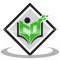## Simplify and solve the following linear equations.$15(y-4)-2(y-9)+5(y+6)=0$.Updated on 10-Oct-2022 13:47:38

## Solve the following linear equation.$m-\frac{m-1}{2}=1-\frac{m-2}{3}$.Updated on 10-Oct-2022 13:47:38
Given:$m-\frac{m-1}{2}=1-\frac{m-2}{3}$.To do:We have to solve the given linear equation.Solution: $m-\frac{m-1}{2}=1-\frac{m-2}{3}$This implies,$m-\frac{m-1}{2}+\frac{m-2}{3}=1$$\frac{6(m) - 3( m-1) +2(m-2)}{6}=1$$6m-3m+3+2m-4= 1\times6$$5m-1= 6$$5m=6+1$$5m=7$$m =\frac{7}{5}$The value of $m$ is $\frac{7}{5}$.

## Solve the following linear equation.$\frac{3 t-2}{4}-\frac{2 t+3}{3}=\frac{2}{3}-t$.Updated on 10-Oct-2022 13:47:38

## Solve the following linear equation.$\frac{n}{2}-\frac{3 n}{4}+\frac{5 n}{6}=21$.Updated on 10-Oct-2022 13:47:38

## Aman's age is three times his son's age. Ten years ago he was five times his son's age. Find their present ages.Updated on 10-Oct-2022 13:47:38
Given :Aman's age is three times his son's age.10 years ago he was five times his son's age.To do :We have to find their present ages.Solution :Let the present age of his son be $x$.This implies, that Aman's present age $=3x$.10 years ago, the age of Aman is 5 times the age of his son.The age of his son 10 years ago $= x-10$The age of Aman 10 years ago $= 3x-10$Therefore, $3x-10=5(x-10)$$3x-10=5x-50$$5x-3x = 50-10$$2x = 40$$x = \frac{40}{2}$$x = 20$$3 x = 3 \times 20 = 60$The present age of Aman is 60 years and the present age of ... Read More

## A grandfather is ten times older than his granddaughter. He is also 54 years older than her. Find their present ages.Updated on 10-Oct-2022 13:47:38
Given:A grand father is ten times older than his granddaughter.He is also 54 years older than her.To do: We have to find the present ages of Grand father and his grand daughter.Solution:Let the present age of the grand daughter be $a$The grand father is ten times older than his granddaughter.This implies, Present age of grand father $= 10a$Grand father is 54 years older than grand daughter. Therefore, Age of grand father $=$ Age of grand daughter $+ 54$$10a = a+54$$10a - a = 54$$9a = 54$$a = \frac{54}{9}$$a=6Therefore, The present age of grand daughter =a$$= 6$ yearsThe present age of grand father ... Read More
Previous 1 ... 4 5 6 7 8 ... 2373 Next